### 4.2.2.3 LEVENBERG-MARQUARDT Algorithm

The LEVENBERG-MARQUARDT algorithm  is an efficient method to solve non-linear least squares problems . Thus, it is well suited for complex inverse modeling tasks especially for TCAD applications where the aim of the LEVENBERG-MARQUARDT algorithm is to optimize (minimize) a twice differentiable function(4.22)

If the original objective function is vector valued, an additional norm has to applied to map the vector to a scalar-valued quantity. The second derivative of the function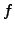is determined by its HESSian4.6 matrix. Because the optimization tasks for TCAD problems cannot be described analytically, the derivatives have to be calculated for each single point. Since there is no guarantee that the HESSian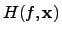is positive definite for non-quadratic forms, the search algorithm might search in the wrong direction. Therefore, a correction term can be introduced to cover this problem by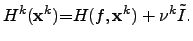(4.23)

If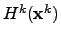is still not positive definite, the factor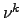is increased by a certain user-defined factor. Sinceis now per definitionem positive definite, the next pointcan be calculated by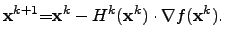(4.24)

However, if there is no improvement in the last minimization step (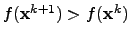), the factorhas to be modified again and the previously described steps have to be recalculated.

This method is a more robust method than the GAUSS-NEWTON method  and provides in general an optimum on less iterations. Nevertheless, if the initial guess ofis too close to the optimal value, the convergence might be slower than that of the GAUSS-NEWTON method.

Stefan Holzer 2007-11-19